Untitled Document

## Predicting mathematics performance from anxiety, enjoyment, value, and self-efficacy beliefs towards mathematics among engineering majors

Dawit Asrat Getahuna1, Getachew Adamub, Awoke Andargieb, Jejaw Demamu Mebratb
a Department of Psychology, College of Education & Behavioral Sciences, Bahir Dar University.
b Department of Mathematics, College of Science, Bahir Dar University.

Abstract: The purpose of this study was to examine first year students’ mathematics anxiety, enjoyment of mathematics, value of mathematics, self-efficacy beliefs and prior performance as predictors of performance on a first year university mathematics course (Applied mathematics I). Data were collected from 225 first year undergraduate students at Bahir Dar University at the beginning and at the end of the course. A rating scale measuring anxiety (11 items), enjoyment of mathematics (14 items), value of mathematics (14 items), mathematics problem solving self-efficacy (9 items) and mathematics tasks self-efficacy (9 items) was used to collect data. The items were adapted from the literature. The participants’ prior performance was assessed by preparing a test from contents of high school mathematics and their performance on the Applied Mathematics course was measured by preparing a test consisting of representative items from the chapters of the course. One-sample t-tests and multiple regression analyses were used to analyze the data. Results revealed that the participants had lower mathematics anxiety, higher level of mathematics enjoyment and value of mathematics. But self-efficacy beliefs were found to be below expected levels. Results from the regression analyses depicted that mathematics enjoyment and mathematics tasks self-efficacy were found to be significant predictors of mathematics problem solving self- efficacy. On the other hand, prior achievement (performance on pre-university mathematics) and self-efficacy beliefs were found to be significant predictors of performance on the university mathematics course. The results imply the need for enhancing students’ self-efficacy beliefs so as to promote their mathematics performance.

Keywords: anxiety, enjoyment, value of mathematics and self-efficacy.

## The problem

Advancement in science and technology is essential for the society to come up with effective solutions to the problems faced. In these days, technology is growing at a very rapid rate. Every year, we see technologies of the preceding years becoming obsolete and substituted by new innovations. Behind this scene of advancement in technology is mathematics, which is believed to be the basis of all fields in the natural as well as the social sciences (AAAS, 1990). Hence, the teaching and learning of mathematics needs to be given due consideration.

In most cases, mathematics is considered a difficult school subject (Countryman, 1992; Sobel & Maletsky, 1999; Van deWalle, 2001). Students’ lower scores are mostly observed in mathematics and mathematics related subjects, compared to other school subjects. For example, observation of students’ grades for the last three years in the course Applied

1 Corresponding author

Mathematics I at Bahir Dar University shows a steady increase in the number of students who score below passing grades. Observation of data for the years 2010, 2011, and 2012 shows that 38%, 53%, and 57% of the students who took the course in the respective years scored below a passing mark of C. (See Table 1)

Table 1 Percentages of grades for the course Applied Mathematics I at BDU for the three years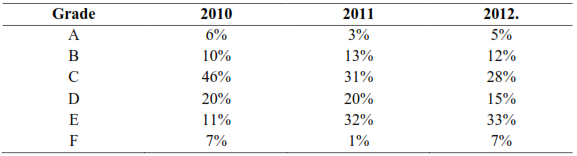Source: Mark lists from course instructors

However, the problem of low achievement may not be due to the inherent difficulty of the subject but it may be related to the way students think about mathematics. What is the anxiety level of students about mathematics? To what extent do students enjoy learning mathematics? To what extent do students believe that mathematics is a worthwhile subject? What is the level of confidence students have in doing mathematics tasks and problems? Such issues are worth considering as they are essential factors for success in mastering mathematics (Ashcraft & Ridley, 2005; Liu & Koirala, 2009; Pinxten, Marsh, De Fraine, Noortgate, & Van Damme; Chouinard, Karsent, and Roy, 2007). Hence, this study was aimed at examining first year undergraduate students’ anxiety, enjoyment, value of mathematics and their self- efficacy in doing mathematics tasks and problems.

Anxiety denotes a feeling of tension towards some events or phenomena (Ashcraft, 2002). Accordingly, mathematics anxiety is a feeling of tension or fear that interferes with individuals’ engagement in manipulating numbers and solving mathematics problems (Hembree, 1990; Kazelskis et al., 2000; Richardson & Suinn, 1972). Mathematics anxiety is claimed to have some influence on students’ attitude towards mathematics, motivation to learn mathematics and subsequently on their mathematics performance. Students who suffer from higher levels of mathematics anxiety typically develop negative attitudes and emotions toward mathematics that will consequently lead to a decreased level of achievement in mathematics. In one study by Cates and Rhymer (2003), students’ level of mathematics anxiety was found to be negatively related to their computational fluency in all areas of mathematical computations. Those students with higher levels of anxiety were found to have lower computational fluency and lower levels of achievement in mathematics. Besides its role in influencing achievement in mathematics, mathematics anxiety influences students’ motivation to learn mathematics (May, 2009). Students with lower level of anxiety toward mathematics are more likely to be motivated to learn mathematics (Middleton & Spanias, 1999) than those with higher levels of anxiety. As mathematics anxiety is so crucial in students’ learning, it is worth examining the status of anxiety among our undergraduate students.

Another factor that may be attributed to low mathematics performance is low level of mathematics enjoyment. Enjoyment denotes the degree to which students enjoy working some tasks, such as mathematics, and the extent to which they are happy in a setting where the task is undergoing, such as mathematics classes (Tapia & Marsh, 2004). It tells about the extent to which students are happy and free from boredom when learning mathematics. It shows the level of motivation students have for learning the subject.

Enjoyment also implies an emotion which is triggered by stimuli is an instinctive or intuitive feeling. Though classroom experiences may be found meaningful and worthwhile, they may not be enjoyable or exciting for learners (Brophy, 1983). Students are likely to demonstrate curiosity to know, motivation to learn, and diligence (perseverance) in doing tasks when they enjoy their learning (Carr, 1997; Lane, 1999; Le seur, 1994).

Literature on the effects of enjoyment on learning and achievement is scant (Schukajlow, et al., 2012). Even the existing studies show contradicting findings. While some studies have found that students’ enjoyment of learning correlates positively with their academic achievement (Pekrun, et al., 2002), others indicated no relationship between achievement and enjoyment (Pekrun, Elliot, & Maier, 2009). Such inconsistent results justify the need for examining the extent to which enjoyment of a subject matter, mathematics in the context of the present study, serves as a predictor of performance. Furthermore, it is worth studying how students’ enjoyment in learning mathematics is related to other psychological variables.

The third possible factor that may influence students’ engagement in learning mathematics is the value they place on mathematics. A value denotes the importance individuals attach to the tasks they engage in (Eccles & Wigfield, 2002). Individuals are more likely to be motivated to engage in tasks they value (Wigfield & Eccles, 1992). Likewise, a belief that mathematics contributes to human civilization and in our everyday life tends to encourage students to provide due consideration to the learning of mathematics. There are claims that students who highly value mathematics are described by teachers as better learners (Metallidou & Vlachou, 2010). Hence, it is a necessity to examine the status of undergraduate students’ value of
mathematics as a factor in their performance.

Finally, self-efficacy belief is another important factor for students’ performance in general and mathematics performance in particular. Self-efficacy refers to individual’s judgement of one’s own ability to perform a task and achieve desired goals (Bandura, 1977). Self-efficacy influences individuals’ choices of the kinds of tasks they would like to do as well as their tendency to persist on difficult tasks (Luszczynska & Schwarzer, 2005).

Mathematics self-efficacy can be commonly defined as individuals’ beliefs or perceptions regarding their abilities in mathematics. In other words, an individual’s mathematics self- efficacy is his or her confidence about completing a variety of tasks, from understanding concepts to solving problems, in mathematics. As it is the case in other domains of tasks, lower self-efficacy in mathematics decreases students’ motivation to learn and subsequently results in lower mathematics achievement (May, 2009). Besides, as self-efficacy plays a major mediational role in students’ task and career related choices, lower mathematics self- efficacy likely leads students to avoid choosing mathematics related careers as well as mathematics related college majors (Hackett & Betz, 1989).

In general, for low achievement in mathematics, there can be a multitude of contributing factors. In the present study, the researchers wanted to examine the extent of undergraduate students’ anxiety, enjoyment, value, and self-efficacy beliefs about mathematics and their contribution in predicting performance in a mathematics course among freshman engineering students at Bahir Dar University. Beyond informing the prevailing situation of the students with regard to the variables of interest, the study has the benefit of giving direction to the kind of intervention that will be meant to improve the mathematics performance of students. Hence, it was aimed to answer the following research questions.

1. What is the extent of first year students’ anxiety, enjoyment, and value of mathematics?
2. What is the extent of first year students’ self-efficacy beliefs in performing mathematics tasks and in solving mathematics problems for pre-university and university (pre-engineering) courses?
3. What is the contribution of affect-related variables (anxiety, enjoyment, and value of mathematics) and students’ self-efficacy beliefs to performing mathematics tasks and to self-efficacy beliefs in solving mathematics problems?
4. What is the contribution of affect-related variables (anxiety, enjoyment, and value of mathematics), self-efficacy beliefs and prior performance to prediction of university mathematics course performance?

## Design of the study

The study is a quantitative survey study meant to examine the contribution of anxiety, enjoyment, value, and self-efficacy beliefs about mathematics to predicting mathematics course performance among first year engineering majors.

## Participants

The participants of the study were first year freshmen engineering students at Bahir Dar University. Freshman engineering students register for a course titled Applied Mathematics I in the first semester of their entry. The course is a basic course for subsequent courses in calculus. In 2013/14 academic year, the total number of the students who were registered for the course was1600 distributed in 19 sections. A random sample of five sections, comprising 350 students, was taken for the study. However, only 270 participants returned the questionnaires distributed. Of these, 225 students responded to all items of the questionnaire.

## Variables in the study

The variables examined in the study were mathematics anxiety, enjoyment of mathematics, value of mathematics, mathematics tasks self-efficacy (coded as MTSE1 for pre-university mathematics and MTSE2 for pre-engineering mathematics course), mathematics problem solving self-efficacy (coded as MPSE1 for pre-university mathematics and MPSE2 for pre- engineering mathematics course), and mathematics performance (coded as Ach1 for pre- university mathematics and Ach2 for pre-engineering mathematics course).

## Instruments

Mathematics Anxiety Scale: This measured mathematics anxiety, using 11 items adapted from items of the scales used in previous studies (Fennema & Sherman, 1976; Kalder & Lesik, 2011). The scale was a five point scale ranging from 1 (strongly disagree) to 5 (strongly agree). While high scores in the scale represent higher level of anxiety, lower scores represent lower level of anxiety. There were six items that needed reverse scoring. Later reliability analysis indicated that the alpha coefficient estimate of reliability for the scale was 0.86.

Mathematics Enjoyment Scale: This assessed enjoyment of mathematics, using 14 items extracted and adapted from Aiken (1974), Kalder and Lesiki (2011) and Tapia and Marsh (2002). Similar to the anxiety scale, it was a five point scale ranging from 1 (strongly disagree) to 5 (strongly agree). Higher scores were made to represent high enjoyment and lower scores to low enjoyment of working mathematics and being in mathematics classes. There were three items which required reverse scoring. The alpha reliability estimate of the scale was found to be 0.92.

Mathematics Value Scale: This was intended to measure the students’ perception of the usefulness of mathematics in everyday life and to the world in general. Fourteen items were adapted from Aiken (1974), Kalder and Lesiki (2011) and Tapia and Marsh (2002) to be used in the scale. Similar to the above scales, it was a five point scale ranging from 1 (strongly disagree) to 5 (strongly agree). Again, here higher scores represented high value and lower scores did low value students give to mathematics. Three of the items required reverse scoring. The alpha reliability estimate of the scale was 0.76.

Mathematics Tasks Self-efficacy Scale: This was meant to measure the students’ confidence in performing different mathematical tasks. There were two versions for this scale: pre-university mathematics tasks (MTSE 1) and pre-engineering mathematics course tasks (MTSE 2). By examining preparatory school textbooks, nine task areas were identified and the students were asked to indicate the extent to which they can perform each task on a 10 point scale ranging from 0 (no confidence at all) to 9 (compete confidence). Similarly, after the completion of the pre-engineering mathematics course, nine task areas were identified and students were asked to rate their level of confidence in performing each task. The alpha reliability estimates for both versions were found to be 0.94.

Mathematics Problem Solving Self-efficacy Scale: This scale was meant to assess the students’ confidence in solving specific mathematics problems derived from the mathematics tasks described in the Mathematics Tasks Self-efficacy scales. Again, this scale had two versions: one for problems derived from pre-university mathematics tasks (MPSE 1) and the other for problems derived from pre-engineering mathematics course tasks (MPSE 2). A problem solving item was constructed for each of the corresponding tasks in the Mathematics Tasks Self-efficacy scales. For both versions, a 10 point scale ranging from 0 (no confidence at all) to 9 (compete confidence) was used. While the alpha reliability estimates for MPSE 1 was 0.95, that of MPSE 2 was 0.93.

Mathematics Performance: The students’ mathematics performances were assessed using the problems used to assess their problem solving self-efficacy. Two performance scores were obtained: one based on their solutions to problems derived from pre-university math tasks (Ach 1) and the second one based on their solutions to problems derived from pre- engineering mathematics course tasks (Ach 2).

## Data collecting procedures

The data collection procedure had two phases. In Phase One, scales designed to measure mathematics anxiety, enjoyment of mathematics, value of mathematics, self-efficacy for pre- university mathematics tasks (MTSE 1), and self-efficacy for solving pre-university mathematics problems (MPSE 1) were administered during the first week of the first semester (2013/14 academic year). In addition to completing the questionnaires, the students were asked to solve the problems used to assess their problem solving self-efficacy. The obtained result was used to represent their background knowledge of mathematics. In Phase Two, after the students took a pre-engineering mathematics course, they completed self- efficacy questionnaires for pre-engineering mathematics tasks (MTSE 2), and for solving pre- engineering mathematics course problems (MPSE 2). Subsequent solutions to the problems were used to represent performance in the course (Ach 2).

## Data Analysis

The study was purely a quantitative study. The first objective of the study was to examine whether the levels of the students’ mathematics anxiety, enjoyment of mathematics, value of mathematics, and self-efficacy beliefs are low or above the desired level (Research Questions 1 and 2). That is to know the overall status of the students’ mathematics anxiety, enjoyment of mathematics, value of mathematics, and their self-efficacy beliefs. Towards this end, a series of one-sample t-tests were applied. The second objective of the study was to examine which variables contribute to the prediction of pre-university mathematics problem solving self-efficacy (MPSE 1) and pre-engineering mathematics course problems self-efficacy (MPSE 2) (Research Questions 3 and 4). Regression analyses were employed by taking math anxiety, mathematics enjoyment, value of mathematics and pre-university mathematics tasks self-efficacy as predictors of MPSE 1 and math anxiety, mathematics enjoyment, value of mathematics and pre-engineering mathematics tasks self-efficacy as predictors of MPSE 2. Finally, the students’ performance on the pre-engineering mathematics course was predicted from math anxiety, mathematics enjoyment, value of mathematics, MTSE 2, and MPSE 2.

## Results

As mentioned earlier, the first objective in the study was to examine the extent to which the students’ mathematics anxiety, enjoyment of mathematics, value of mathematics, and self- efficacy beliefs are at the desired level of occurrences. This was done by running one sample t-tests for each of the variables. Following are the results of the analyses.

## Mathematics anxiety

Before running one-sample t-test analysis, missing values were examined. Initially, four cases were removed as the participants did not complete at least 50% of the items of the scale. Furthermore, as 13% of the entries for Item 10 of the scale were missing, the item was removed. The remaining missing values were substituted using the variable (item) means. In this scale, higher scores indicate high level of anxiety and lower scores indicate low level of anxiety. A response of agree (a rating of 4) was taken as an expected value to infer about the anxiety level of the students. That is, in order to say that a student has a high level of anxiety, he/she has to respond to an item as agree or above. On the basis of this, the expected total score was set to be at 40 (since there are 10 items in the scale, rating each of these as 4 will result in a total score of 40). Comparison of the obtained mean anxiety score (25.50) against the expected value (40) revealed that the obtained mean anxiety score is significantly below the expected value. This informs that the students’ mathematics anxiety is low. Table 2 shows the one-sample t-test result for mathematics anxiety.

Table 2 One-Sample t-test Results for Math Anxiety Scale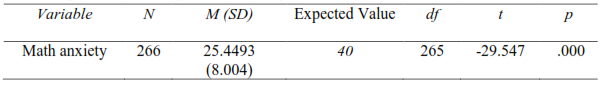## Enjoyment of mathematics

Only one case, out of the 270 participants, was removed because of many (more than 10%) incomplete data. Other missing values were substituted using variable mean substitution method. As there were 14 items in this scale, the testing value was set to be 56 in order to run the one-sample t-test. High scores in this scale represent high enjoyment; low scores, low enjoyment. In order to say that a student has really enjoyed mathematics, he/she has to at least provide a response of agree in the scale – which was represented by a rating value of 4. Hence, the summation score set as an expected value was 56 (14 items X 4). As shown in Table 3, the obtained mean value (56.57) was not significantly different from the expected value indicating that the students do really enjoy mathematics at the expected level.

Table 3 One-Sample t-test Results for Math Enjoyment Scale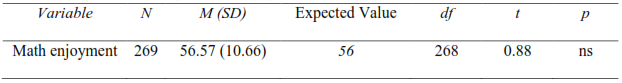## Value mathematics

One case with considerable missing data was removed. Other missing values were substituted by variable means. Alpha coefficient of reliability was found to be 0.76. Similar to the enjoyment scale, there were 14 items in this scale and higher scores indicate high value and lower scores do low value. In the same way, a response of agree was taken as an expected response. That is, if a student really values mathematics, he/she has to at least agree with each statement, which was represented by a rating of 4. Thus, a total score of 56 was taken as a testing value. As can be seen from Table 4, the students valued mathematics significantly higher than the expected value.

Table 4 One-Sample t-test Results for Math Value Scale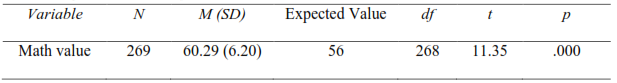Out of 270 participants who were given the instruments, 245 judged their self-efficacy for the tasks identified for pre-university mathematics. For missing values, variable means were substituted. The alpha reliability coefficient of the scale was found to be 0.94. The scale was intended to examine the extent to which students are confident in performing different mathematics tasks which are the focus of pre-university mathematics. This was done using a 10-point scale ranging from 0 to 9. To determine the testing value, first, the range of the scores in the scale was divided into three equal sections. The cut-off point dividing the bottom-third and the middle-third sections was 3.33 and the one dividing the middle-third and the top-third was 6.66. These values were obtained by dividing 10 by three. The divisions of bottom-third, middle-third and top-third respectively represented low confidence, medium confidence, and high confidence in pre-university math tasks. Based on this, to claim that a student has confidence in the tasks, he/she has to have an average rating of 6.66 or above or a total score of 60 (6.6 X 9 items) or above. Hence, the testing value was set at 60. As indicated in Table 5, the students’ self-efficacy in the pre-university mathematics tasks was considerably below the expected indicating low self-efficacy.

Table 5 One-Sample t-test Results for Pre-university Math Tasks Self-efficacy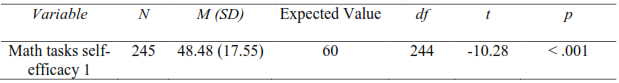## Pre-university math problem solving self-efficacy

By preparing mathematics problems corresponding to each of the mathematics tasks included in the measure of pre-university math tasks self-efficacy, the students were asked to rate the extent to which they are confident to solve the problems. For this scale, there were 254 respondents. Missing values were substituted by variable means. The alpha reliability coefficient of the scale was found to be 0.95. To run the one-sample t-test for this case, similar procedures and justifications to the case for pre-university math tasks self-efficacy were used. The result of the analysis, as shown in Table 6, indicated that the students’ self- efficacy to solve pre-university math problems was considerably lower than expectation.

Table 6 One-Sample t-test Results for Pre-university Math Problems Self-efficacy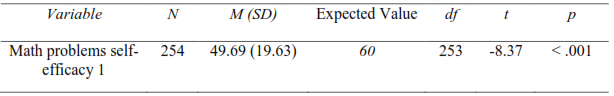After the participants completed a one semester pre-engineering math course, the tasks covered in the course were identified and they were asked to rate their confidence in performing the tasks. Out of 270 participants, 259 completed the questionnaire. But eight of these respondents did not give complete responses; consequently, they were removed from subsequent analyses. For the remaining 251 cases, missing values were substituted by variable means. Alpha reliability coefficient was found to be 0.94. The same procedures and justifications as the case for pre-university math tasks and math problems self-efficacy scales were used to run the one-sample t-test. The result indicated that the students’ self-efficacy to perform the tasks covered in the pre-engineering math course was considerably lower than expected.

Table 7 One-Sample t-test Results for Pre-engineering Math Course Tasks Self-efficacy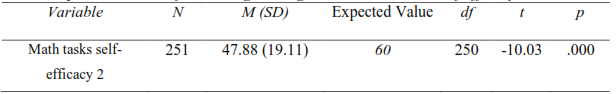## Pre-engineering math problems self-efficacy

Mathematics problems corresponding to each of the mathematics tasks included in the measure of the pre-engineering math tasks self-efficacy were prepared. The participants were asked to rate their confidence to solve them. Two hundred forty rated their confidence. The missing values were substituted by variable means. The alpha reliability coefficient was found to be 0.93. One-sample t-test result indicated that the students’ confidence was in general considerably lower than the expected level.

Table 8 One-Sample t-test Results for Pre-engineering Math Course Tasks Self-efficacy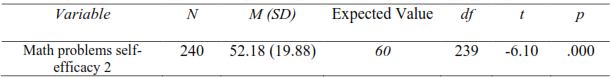## Results from Regression Analyses

Three sets of regression analyses were conducted. Two of them were intended to see predictors of mathematics problem solving self-efficacy (separately for pre-university math (MPSE1) and pre-engineering math course (MPSE2)) and the third regression was meant to predict performance on pre-engineering math course from sets of factors. Table 9 shows the bivariate correlations of the variables involved in the three sets of regression analyses.

Table 9 Summary statistics for correlations among variables, variable means, and standard deviations.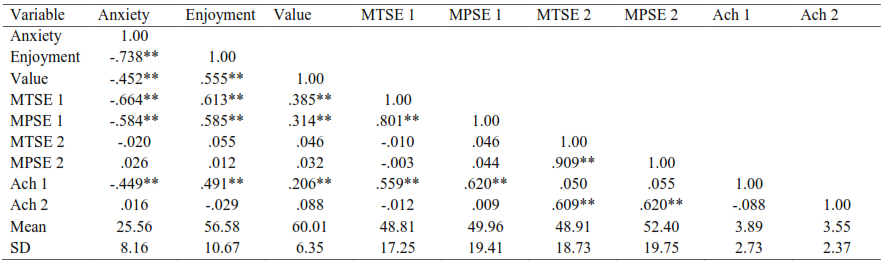MTSE 1= pre-university mathematics tasks self-efficacy   MTSE 2= pre-engineering mathematics tasks self-efficacy
MPSE 1= pre-university mathematics problem solving self-efficacy   MPSE 2= pre-engineering mathematics problem solving self-efficacy
Ach 1 = performance on pre-university math problems   Ach 2 = performance on pre-engineering math problems

## 1. Prediction of pre-university math problems self-efficacy (MPSE 1)

In order to know what factor(s) contribute for the participants’ confidence in solving mathematics problems from their pre-university courses, a regression analysis was conducted by taking math anxiety, enjoyment of math, value of math, and math tasks self-efficacy for pre-university math as predictors. Collinearity diagnosis was conducted by looking at Variance Inflation Factor (VIF) values as well as Condition Index (CI) and Variance Proportion (VP) values. From these values it was evident that there was no problem of collinearity.

The number of complete cases for all of the five variables was 225. The regression analysis revealed that enjoyment of math and math tasks self-efficacy were the only significant predictors for the students’ confidence in solving pre-university math problems (Table 10).

Table 10 Regression Analysis of Predictors on Pre-university Math Problems Self-efficacy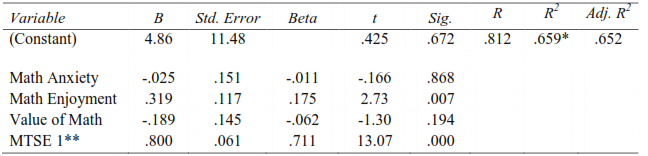Dependent Variable: pre-university math problems self-efficacy (MPSE 1); * p < .001 ** Pre-university math tasks self-efficacy

## 2. Predictors of pre-engineering math course problems self-efficacy (MPSE 2)

To know the factor(s) that contribute for the participants’ confidence in solving mathematics problems from their pre-engineering course, a regression analysis was conducted by taking math anxiety, enjoyment of math, value of math, and math tasks self-efficacy for pre- engineering math course as predictors. The VIF, CI and VP values indicated that there was no problem of collinearlity.

Similar to the previous regression analysis, the number of complete cases for all of the five variables was 225. The regression analysis revealed that only pre-engineering math tasks self- efficacy (MTSE 2) was the significant predictor for the students’ confidence in solving pre- engineering math course problems (Tables 11).

Table 11 Regression Analysis of Predictors on Pre-engineering Math Course Problems Self-efficacyDependent Variable: pre-engineering math course problems self-efficacy (MPSE 2); *p<.001 ** Pre-engineering math course tasks self-efficacy

## 3. Predictors of pre-engineering course performance

In order to determine the factors that contribute for the participants’ performance on pre- engineering course problems, a third regression analysis was conducted. The predictor variables included were: math anxiety, enjoyment of math, value of math, pre-engineering math course tasks self-efficacy, pre-engineering math course problem solving self-efficacy, and pre-university math problems performance. The performances were assessed using the problems presented to judge their self-efficacy beliefs. That is, after asking the students to rate the extent of their confidence in solving the problems, they were asked to solve each of them. While solutions to the pre-university math problems were taken to represent pre- university math problems performance, those to pre-engineering math course problems were taken to represent pre-engineering math course performance.

Results from the regression analysis revealed pre-university math problems performance, value of math, pre-engineering math course tasks self-efficacy, and pre-engineering math course problem solving self-efficacy as significant predictors. But math anxiety and math enjoyment were not significant predictors of performance as the results of the analysis shown in Tables 12 below.

Table 12 Regression Analysis of Predictors on Pre-engineering Math Problems PerformanceDependent Variable: pre-engineering math problems performance (Ach 2); *p<.001

## Discussion and Implications

The results in general indicated that students had overall lower level of math anxiety, high value to the contribution of math, and high enjoyment of math. The correlation results indicated that math anxiety was significantly and negatively related to all other variables in the first phase of the study (enjoyment, value, MTSE 1, MPSE 1, and Ach 1). Such a result was also evident in studies which examined math anxiety in relation to performance (Pourmoslemi, Erfani, andFiroozfar, 2013), self-efficacy (Meece, Wigfield, and Eccels, 1990), and perception of importance of math (Meece et al., 1990; Joseph, 2005; Sherman and Wither, 2003). However, anxiety was not related to the variables in the second phase of the study (MTSE 2, MPSE 2 and Ach 2). This can be due to the contextual nature of variables involved. The MTSE 2 and the MPSE 2 represent self-efficacy beliefs towards tasks and problems which are different from those used to assess MTSE 1 and MPSE 1. There is very high likelihood that the students took into account pre-university mathematics as a reference point in rating their level of anxiety. They would probably have taken into account pre- engineering mathematics if there had been a second round assessment of their level of anxiety.

The high value of math may emanate from the students’ awareness of the emphasis given to science disciplines in the education system in general and the very essential role math plays in the disciplines. Currently, 70% of students who have attended preparatory schools are required to pursue their university studies in the fields of science and engineering. Mathematics is a compulsory subject for these fields of studies. This might have instigated them to think that mathematics is a very valuable subject area. The enjoyment they expressed of math also corresponds with this result. The considerable level of overall enjoyment of math the students revealed may be due to the perceived value of math by the participants. Enjoyment denotes the degree to which students enjoy working mathematics and mathematics classes (Tapia & Marsh, 2004). The enjoyment may also be a reflection of the methodology of teaching being exercised currently at schools and universities. Nowadays, at various school levels (including higher education), peer groups are organized for collaborative learning which may be helping students develop a sense of cooperation. This might have served as a source of their enjoyment.

Math self-efficacy (both task and problem solving self-efficacy) scores indicated that the students have below expected levels of confidence in doing math tasks and solving math problems. Given the high value of math as a subject and considerable enjoyment of it, it seems paradoxical for the students demonstrating overall low level of confidence. This can be explained in terms of the nature of measures used for the attributes. In case of the measures of value and enjoyment, the items used were broader and less contextualized than items meant to measure self-efficacy. In contrast, the self-efficacy measuring items presented to the students were specific types of tasks and specific problems upon which the students can specifically judge their level of confidence. However, regardless of the fact that the overall level of confidence is low, still there are significant positive correlations among the dimensions of self-efficacy beliefs (math tasks self-efficacy and math problems self-efficacy) and math value and enjoyment. Students with relatively better self-efficacy were found to value and enjoy better math.

Results of the regression analyses revealed math tasks self-efficacy, together with math enjoyment, as a significant predictor of pre-university math problem solving self-efficacy and a sole significant predictor of the pre-engineering math problem solving self-efficacy. In both analyses, it has been found that general confidence in doing math tasks significantly predicted confidence to solve specific problems generated from the tasks. The significance of math enjoyment as a predictor for solving pre-university math problems but not pre-engineering math problems can be explained in terms of the context the participants might have considered when responding to the enjoyment items. The enjoyment scale was administered at the beginning together with pre-university math tasks and math problem solving self- efficacy scales. When responding to the enjoyment scale, what might have come in the participants’ thinking are the pre-university math courses. That is, they might have expressed their enjoyment of math in the context of the pre-university math. The enjoyment of math reported in such a context might not be representative of enjoyment on a pre-engineering math course and hence may not predict problem solving self-efficacy on the course. If their enjoyment had also been examined after they took the pre-engineering course, it would possibly have been a significant predictor.

The study revealed prior performance and self-efficacy factors (both math tasks self-efficacy and math problem solving self-efficacy) as significant predictors of performance in the pre- engineering course. There are claims about the importance of prior knowledge and performance for subsequent performance. According to the constructivist perspective, learners’ prior learning and experiences are essential to develop new concepts (Beck & Kosnik, 2006; Clements & Battista, 1990). Hence, the fact that the tasks at pre-university math are essential prerequisites for the pre-engineering math topics might have contributed to their prior performance’s (achievement on pre-university math) significant prediction of their later performance (achievement on the pre-engineering course). Besides the participants’ prior achievement, and both math tasks self-efficacy and math problem solving self-efficacy had significant contributions to the prediction of performance in pre-engineering course. This is consistent with what a number of prior studies (Pajares & Miller, 1994, 1995) have documented about the role self-efficacy plays in students’ performance.

In general, the study revealed that, rather than factors related to affective variable (anxiety, enjoyment, and value), cognitive related variables (self-efficacy) and background related variable (prior achievement) are important predictors of subsequent performance. The implication is that it is worth working on enhancing students’ sense of confidence in doing tasks so as to promote their performance. So far, efforts to improve students’ performance in general, and mathematics performance in particular, focused on academic based interventions such as giving tutorials or extra worksheets. Future interventions should go further and work on the psychological aspects particularly on mathematics self-efficacy beliefs of students.

## References

• Aiken, L. R. (1974). Two scales of attitude toward Mathematics. Journal for Research in Mathematics Education, 5(2), 67-71
• American Association for the Advancement of Science. (1990). Project 2061: Science for all Americans. New York: Oxford University Press.
• Ashcraft, M. H. (2002). Math anxiety: Personal, educational, and cognitive consequences. Directions in psychological Science, 11, 181-185
• Ashcraft, M. H., & Ridley, K. S. (2005). Math anxiety and its cognitive consequences: A tutorial review. In J. I. D. Campbell (Ed.).Handbook of mathematical cognition, (pp. 315 ¢ 330). New York: Psychology Press
• Bandura, A. (1977). Self-efficacy: toward a unifying theory of behavioral change. Psychological Review, 84(2), 191-215.
• Beck, C. and Kosnik, C. (2006). Innovations in teacher education: A social constructivist approach. New York: State University of New York Press.
• Betz, N. E., & Hackett, G. (1983). The relationship of mathematics self-efficacy expectations to the selection of science-based college majors. Journal of Vocational Behavior, 23, 329345.
• Brophy, J. (1983). Fostering student learning and motivation in the elementary school classroom. In S. G. Paris, V. Sarris, A. Parducci, G. M. Olson, and H. W. Stevenson (Eds). Learning and motivation in the classroom (pp. 283 ¢ 305), NJ: Lawrence Erlbaum
• Carr, M. (1997). Persistence when it is difficult: A disposition to learn for early childhood. Earlychild hood folio, 3, 9-12
• Cates, G. L., & Rhymer, K. N. (2003). Examining the relationship between mathematics anxiety and mathematics performance: An instructional hierarchy perspective. Journal of Behavioral Education, 12, 23-34
• Chouinard, R., Karsent, J., & Roy, N. (2007). Relations among competence beliefs, utility value, achievement goals, and effort in mathematics. British Journal of Educational Psychology, 77, 501 ¢ 517
• Clements, D. H., & Battista, M. T. (1990). Constructivist learning and teaching. The Arithmetic Teacher, 38(1), 34-35.
• Countryman, J. (1992). Writing to learn mathematics: Methods that work. Portsmouth: Heinemann.
• Eccles, J., & Wigfield, A. (2002). Motivational beliefs, values and goals. Annual Review of Psychology. 53, 109¢32.
• Fennema, E., & Sherman, J. A. (1976). Fennema-Sherman mathematics attitudes scales; instruments desinged to measure attitudes towards the learning of mathematics by females and males. Catalog of Selected Documents in Psychology, 6(1), 31.
• Hackett, G., & Betz, N. E. (1989). An Exploration of the Mathematics SelfEfficacy/Mathematics PerformanceCorrespondence. Journal for Research in Mathematics Education, 20(3), 261 - 273
• Hembree, R. (1990). The nature, effects, and relief of mathematics anxiety. Journal of Research in Mathematics Education, 21, 33-46
• Joseph, Y. K. K. (2005). Anxiety and performance on mathematical problem solving of secondary two students in Singapore. The Mathematics Educator, 8(2), 71-83
• Kalder, R. S., & Lesik, S. A. (2011). A classification of attitudes and beliefs towards mathematics for secondary mathematics pre-service teachers and elementary preservice teachers: An exploratory study using latent class analysis. Issues in the Undergraduate Mathematics Preparation of School Teachers, 5, 593 ¢ 611
• Kazelskis, R., Reeves, C., Kersh, M. E., Bailey, G., Cole, K., Larmon, M., . . . Holliday, D. C. (2000). Mathematics Anxiety and Test Anxiety: Separate Constructs? The Journal of Experimental Education, 68(2), 137-146
• Lane, R. (1999). A model for teaching and learning mathematics. Paper presented at the sixth biennial conference of the New Zealand Association of Mathematics Teachers, Dunedin, New Zealand
• Liu, X., & Koirala, H. (2005). The effect of mathematics self-efficacy on mathematics achievement of high school students. Paper presented at Northeastern Educational Research Association (NERA)
• Luszczynska, A., & Schwarzer, R. (2005). Social cognitive theory. In M. Conner and P. Norman (Eds.), Predicting health behavior (3 rd ed) (pp. 225 ¢ 251). Buckingham: Open University Press
• May, D. K. (2009). Mathematics self efficacy and anxiety questionnaire. Unpublished doctoral dissertation, University of Georgia, Athens, USA
• Meece, J. L., Wigfield, A., & Eccles, J. S. (1990). Predictors of math anxiety and its influence on young adolescents' course enrollment intentions and performance in mathematics. Journal of Educational Psychology, 82(1), 60-70
• Metallidou, P., & Vlachou, A. (2010). ChildrenÆs self-regulated learning profile in language and mathematics: The role of task value beliefs. Psychology in the Schools, 47, 776 ¢ 788
• Middleton, J. A., & Spanias, P. A. (1999). Motivation for achievement in mathematics: Findings, generalizations, and criticisms of the research. Journal for Research in Mathematics Education, 30, 65-88
• Ormrod, J. E. (2006). Educational psychology: Developing learners(5 th ed). New Jersey: Pearson
• Pajares, F., & Miller, M. D. (1994). Role of self-efficacy and self-concept beliefs in mathematical problem solving: a path analysis. Journal of Educational Psychology, 86(2), 193¢203.
• Pajares, F., & Miller, M. D. (1995). Mathematics self-efficacy and mathematics performances: The need for specificity of assessment. Journal of Counseling Psychology, 42(2), 190 - 198
• Pekrun, R., Elliot, A. J., & Maier, M. A. (2009). Achievement goals and achievement emotions: Testing a model of their joint relations with academic performance. Journal of Educational Psychology, 101, 115 ¢ 135.
• Pekrun, R., Goetz, T., Titz, W., & Perry, R. P. (2002). Academic emotions in studentsÆ selfregulated learning and achievement: A program of quantitative and qualitative research. Educational Psychologist, 37, 91 ¢ 106.
• Pinxten, M., Marsh, H. W., De Fraine, B., Noortgate, W. V. D., & Van Damme, J. (2014). Enjoying mathematics or feeling competent in mathematics? Reciprocal effects on mathematics achievement and perceived math effort expenditure. British Journal of Educational Psychology, 84, 152 ¢ 174
• Pourmoslemi, A., Erfani, N., & Firoozfar, I. (2013). Mathematics anxiety, mathematics performance and gender differences among undergraduate students. International Journal of Scientific and Research Publications, 3(7), 1-6
• Richardson, R. C., & Suinn, R. M. (1972). The mathematics anxiety rating scale: Psychometric data. Journal of Counselling Psychology, 19, 551-554
• Schukajlow, S., Leiss, D., Pekrun, R., Blum, W., M³ller M., & Messner R. (2012). Teaching methods for modelling problems and students' task-specific enjoyment, value, interest and self-efficacy expectations. Educational Studies in Mathematics, 79(2),
• Sherman, B. F., & Wither, D. P. (2003). Mathematics anxiety and mathematics achievement. Mathematics Education Research Journal, 15(2), 138-150
• Sobel, M.A., & Maletsky, E.M. (1999). Teaching mathematics : A sourcebook of aids, activities, methods. Boston: Allyn and Bacon.
• Tapia, M., & Marsh, G. E. (2002). Confirmatory factor analysis of the attitudes toward Mathematics inventory. Paper presented at the Annual Meeting of the Mid-South Educational Research Association, Chattanooga, TN (November 6-8)
• Tapia, M., & Marsh, G. E. (2004). An instrument to measure mathematics attitudes. Academic Exchange Quarterly, 8(2), 16-21
• Van de Walle, J. A. (2001). Elementary and middle school mathematics. New York: Longman.
• Wigfield, A., & Eccles, J. (1992). The development of achievement task values: A theoretical analysis. Developmental Review, 12, 265 ¢ 310.

### Refbacks

• There are currently no refbacks.

Copyright (c) 2017 Bahir Dar Journal of Education